×#### Thank you for registering.

One of our academic counsellors will contact you within 1 working day.

Click to Chat

1800-1023-196

+91-120-4616500

CART 0

• 0

MY CART (5)

Use Coupon: CART20 and get 20% off on all online Study Material

ITEM
DETAILS
MRP
DISCOUNT
FINAL PRICE
Total Price: Rs.

There are no items in this cart.
Continue Shopping• Complete JEE Main/Advanced Course and Test Series
• OFFERED PRICE: Rs. 15,900
• View Details

```Revision Notes on Decimals

Decimals

When we use dots to write some numbers then that dot is the decimal point. This is used to show the part of a whole number.Tenths

As we know that 1 cm = 10 mm, so if we have to find the opposite then

1mm = 1/10 cm or one-tenth cm or 0.1 cm.

Hence, the first number after the decimal represents the tenth part of the whole.This reads as “thirty-four point seven”.

Representation of Decimals on Number Line

To represent decimals on the number line we have to divide the gap of each number into 10 equal parts as the decimal shows the tenth part of the number.

Example

Show 0.3, 0.5 and 0.8 on the number line.

Solution

All the three numbers are greater than 0 and less than 1.so we have to make a number line with 0 and 1 and divide the gap into 10 equal parts.

Then mark as shown below.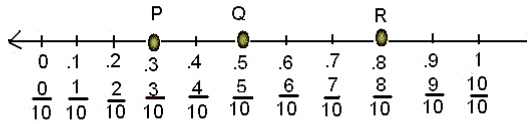Fractions as Decimals

It is easy to write the fractions with 10 as the denominator in decimal form but if the denominator is not 10 then we have to find the equivalent fraction with denominator 10.

Example

Convert 12/5 and 3/2 in decimal form.

SolutionDecimals as Fractions

Example

Write 2.5 in a fraction.

Solution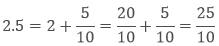Hundredths

As we know that 1 m = 100 cm, so if we have to find the opposite then

1 cm = 1/100 m or one-hundredth m or 0.01 m.

Hence, the second numbers after the decimal represent the hundredth part of the whole.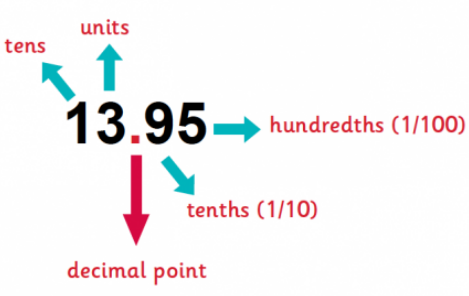It reads as “thirteen point nine five”.

Decimal in the hundredth form shows that we have divided the number into hundred equal parts.

Example

If we say that 25 out of 100 squares are shaded then how will we write it in fraction and decimal form?Solution

25 is a part of 100, so the fraction will be 25/100.

In the decimal form we will write it as 0.25.

Place Value Chart

This is the place value chart which tells the place value of each digit in the decimal number. It makes it easy to write numbers in decimal form.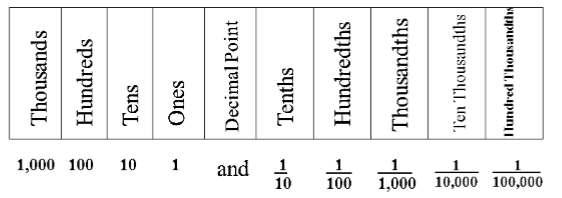Example

With the given place value chart write the number in the decimal form.

Hundreds (100)
Tens (10)
Ones (1)
Tenths (1/10)
Hundredths (1/100)

4
6
3
8
5

Solution

According to the above table-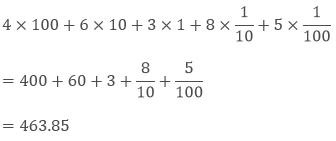Comparing Decimals

1. If the whole number is different.

If the whole numbers of the decimals are different then we can easily compare them .the number with the greater whole number will be greater than the other.

Example

Compare 532.48 and 682.26.

Solution:

As the whole numbers are different, so we can easily find that the number with a greater whole number is greater.

Hence 682.26 > 532.48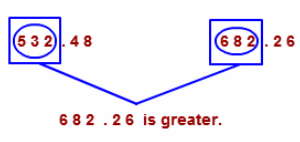2. If the whole number is the same

If the whole numbers of the decimals are same then we will compare the tenth and then the hundredth part if required.

The number with the greater tenth number is greater than the other.

Example

Compare 42.36 and 42.68.

Solution

As the whole number is the same in both the numbers so we have to compare the tenth part.

Hence 42.68 > 42.36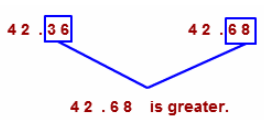Using Decimals

Generally, decimals are used in money, length and weight.

1. Money

Example: 1

Write 25 paise in decimals.

Solution:

100 paise = 1 Rs.

1 paise = 1/100 Rs. = 0.01 Rs.

25 paise = 25/100 Rs. = 0.25 Rs.

Example: 2

Write 7 rupees and 35 paise in decimals.

Solution:

7 rupees is the whole number, so

7 + 35/100 = 7 + 0.35 = 7.35 Rs.

2. Length

Example

If the height of Rani is 175 cm then what will be her height in meters?

Solution

100 cm = 1 m

1 cm = 1/100 m = 0.01 m

175 cm = 175/100 mHence, the height of Rani is 1.75 m.

3. Weight

Example

If the weight of a rice box is 4725 gram then what will be its weight in a kilogram?

Solution

1000 gm = 1 kg

1 gm = 1/1000 kg = 0.001 kg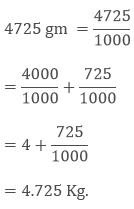To add the decimal numbers we can add them as whole numbers but the decimal will remain at the same place as it was in the given numbers. It means that we have to line up the decimal point in each number while writing them, and then add them as a whole number.

Example: 1

Solution:

Write the numbers as given below, and then add them.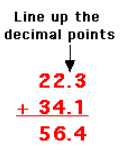Example: 2

Solution:

There are three numbers after decimal in one number and one number after decimal in another number. So we should not get confused and write the numbers by lining up the decimal points of both the numbers, then add them.Another way is to write the numbers in the place value chart, so that it will be easy to identify, how to write numbers.

Ones (1)
Tenths (1/10)
Hundredths (1/100)
Thousandths (1/1000)

+
4
1
0
0

=
5
3
3
4

Subtraction of Decimal Numbers

Subtraction is also done as normal whole numbers after lining up the decimals of the given number.

Example

Subtract 243.86 from 402.10.

Solution

Write the numbers in a line so that the decimal points of both the numbers lined up.

Then subtract and borrow as we do in whole numbers.

Line up the decimal point in the answer also.```### Course Features

• 728 Video Lectures
• Revision Notes
• Previous Year Papers
• Mind Map
• Study Planner
• NCERT Solutions
• Discussion Forum
• Test paper with Video Solution